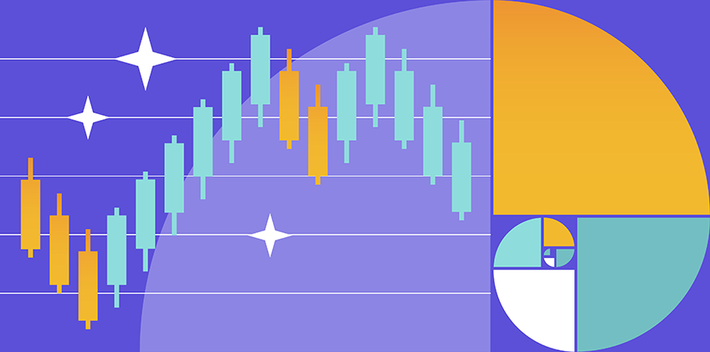# How to Use Fibonacci Retracements?Publication date is

Fibonacci retracement and extension analysis helps traders locate support and resistance levels on asset price charts. Learn how to use Fibonacci retracements to make profitable trades in this article.

## What is Fibonacci Retracements?

Fibonacci retracements is a technical indicator used to identify temporary reversals within an overarching price trend. Fibonacci retracements split the vertical distance between two extreme price points on a chart into key Fibonacci ratios (23.6%, 38.2%, 50%, 61.8%,100%) to indicate levels where a price retracement is likely to occur.

Fibonacci retracement levels are considered a predictive technical indicator as they are designed to forecast future changes in the market.

## How are Fibonacci Ratios Calculated?

Fibonacci sequence ( 0, 1, 1, 2, 3, 5, 8, 13, 21, 34, 55,... ) is a set of integers, where each number is equal to the sum of the preceding two. Fibonacci ratios are derived from mathematical relationships between the numbers in this sequence.

For example:

• The 61.8% Fibonacci ratio is found by dividing one number in the series by the number that follows it.
• 38.2% is the result of dividing a number in the sequence by the number located two places to the right.
• The 23.6% ratio is found by dividing one number in the series by the number that is three places to the right.

## What are Fibonacci Extensions?

While Fibonacci retracements use Fibonacci ratios to measure the price pullbacks within a trend, Fibonacci extensions evaluate how far the asset price will travel in the trending direction after experiencing a retracement. Fibonacci extensions are commonly used as take-profit targets since they are an indicator that the prevailing trend is about to temporarily reverse. The most widely used Fibonacci extensions ratios are: 123.60%, 161.8%, 200%, and 261.8%

## How Fibonacci Retracements Work?

A retracement is a short-term price correction during an overall larger upward or downward trend. Retracements do not indicate a significant change in the market but rather signify a temporary setback in buying/selling pressure.

Fibonacci retracements is a tool that allows traders to identify price points, where a retracement is likely to occur. These price points also commonly coincide with support and resistance levels

Fibonacci retracements take two extreme price points on a chart and split the vertical distance between them into key Fibonacci ratios. They then draw a horizontal line for each level, denoting possible support and resistance levels.

## How to trade Fibonacci Retracements?

1. Find a strong upward or downward movement of a given financial instrument.
2. Identify swing high and swing low points of the trend.
3. Using the Fibonacci retracement tool, draw a line between a trend’s swing low and a swing high.
4. The horizontal lines that are calculated and drawn automatically by the Fibonacci retracement tool will denote possible trend retracement levels.

In the TabTrader app you will find a wide spectrum of advanced tools for technical analysis including Fibonacci retracements. Track and analyze 20K+ real-time updated trading instruments on 30+ major exchanges with our free cryptoсurrency trading terminal.

## How To Draw a Fibonacci Retracement Correctly?

Fibonacci retracements are drawn between two significant points on a chart (usually an absolute low and an absolute high).

## Fibonacci retracements pros and cons

Pros:

• Accuracy. With the correct setting, Fibonacci retracements are often able to quite accurately determine trend retracements in financial instruments.
• Versatility Fibonacci retracements can be used on assets of any market and with any timeframe.
• Ability to predict market moves. Fibonacci retracements are a predictive indicator.

Cons:

• Can not be relied on exclusively. Fibonacci retracements are most effective when combined with additional trading signals such as other indicators and technical chart patterns.
• No logical proof. The underlying principle of any fibonacci tool is a numerical anomaly that is not grounded by any logical proof.

## Conclusion

Extension and retracement Fibonacci levels are proven to be quite effective in signaling possible areas of importance on asset price charts. However it is important to remember that as with any other technical indicator Fibonacci retracements are most reliable when combined with other trading signals.

## FAQ

##### What is the difference between Fibonacci retracements and Fibonacci extensions?

While Fibonacci retracements measure the pullbacks within a price trend, Fibonacci extensions estimate how far the asset price will travel in the trending direction after experiencing a retracement.

##### What do Fibonacci retracements tell you?

Fibonacci retracements indicate possible locations of support and resistance levels on a price chart.

##### Why do Fibonacci retracements work?

At the heart of any Fibonacci instrument is a numerical anomaly, currently unsupported by any logical proof.

##### How do Fibonacci retracements work?

Fibonacci retracements split the vertical distance between two extreme price points on a chart into key Fibonacci ratios (23.6%, 38.2%, 50%, 61.8%,100%) to indicate levels where a price retracement is likely to occur.

Looking to get started in crypto trading?

Try TabTrader on mobile or web!